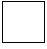Skill 2D
Counting Coins: pennies, nickels, dimes, quarters

While students should easily be able to identify the value of the coins shown, we now jump to the more difficult skill of having students transfer this value to other coins. So in the first example, we ask the student to tell us how many pennies equals the value of 2 quarters. We do show the coins which simplifies the process somewhat.

 1.2 quarters =pennies

2.5 dimes =quarters